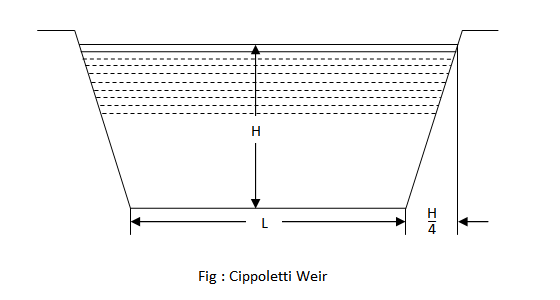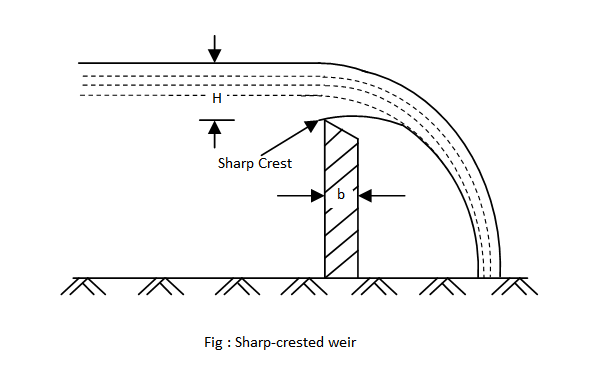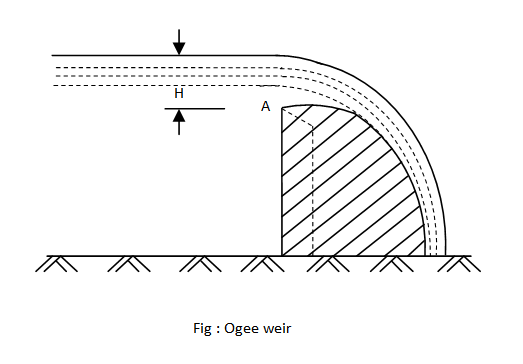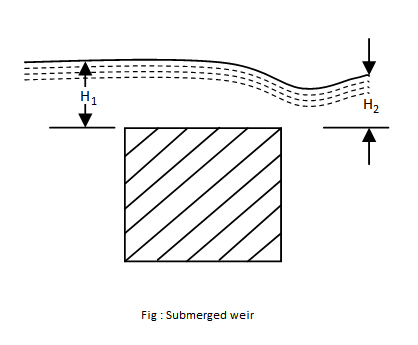I have forgotten
my Password

Or login with:

•http://facebook.com/
•https://www.google.com/accounts/o8/id
•https://me.yahoo.com

# Discharge

Discharge over different types of weirsView version details

### Key Facts

Gyroscopic Couple: The rate of change of angular momentum () =(In the limit).
•= Moment of Inertia.
•= Angular velocity
•= Angular velocity of precession.

Blaise Pascal (1623-1662) was a French mathematician, physicist, inventor, writer and Catholic philosopher.

Leonhard Euler (1707-1783) was a pioneering Swiss mathematician and physicist.

## Discharge Over A Rectangular Weir

Consider a rectangular weir over which the water is flowing as shown in figure -Let,
• H = Height of the water above the crest of the weir
• L = Length of the weir and
• Cd = Coefficient of discharge

Let us consider a horizontal strip of water of thicknessat a depthfrom the water surface as shown in

figure.Area of the strip =We know that the theoretical velocity of water through the strip =Discharge through the strip,=Area of stripTheoretical VelocityThe total discharge, over the weir, may be found out by integrating the above equation within the limits 0 and H.Example:
[metric]
##### Example - Discharge Over A Rectangular Weir
Problem
A weir of 8m long is to be built across a rectangular channel to discharge a flow of 9m3 /s. If the maximum depth of water on the upstream side of weir is to be 2m, what should be the height of the weir ? Adopt Cd = 0.62.
Workings
Given,
• L = 8 m
• Q = 9 m3 /s
• Depth of water = 2m
• Cd = 0.62

Let, H = Height of water above the sill of the weir.

So, the discharge over the weir,

Therefore height of weir should be = 2.0 - 0.72 = 1.28 m
Solution
Height of weir = 1.28 m

## Discharge Over A Cippoletti Weir

The "Cippoletti" weir is a trapezoidal weir, having 1 horizontal to 4 vertical side slopes, as shown in figure. The purpose of the slope, on the sides, is to obtain an increased discharge through the triangular portions of the weir, which, otherwise would have been decreased due to end contractions in the case of rectangular weirs. Thus the advantage of a Cippoletti weir is that the factor of end contraction is not required, while using the Francis' Formula.Let us split up the trapezoidal weir into a rectangular weir and a triangular notch.

Now discharge over a rectangular weir,and discharge over a triangular notchSo total discharge,Since the main idea of Cippoletti was to avoid the factor of end contraction, and as such he gave the formula for the

discharge,Example:
[metric]
##### Example - Discharge Over A Cippoletti Weir
Problem
Water is flowing over a Cippoletti weir of 4 meters long under a head of 1 meter. Compute the discharge, if the coefficient of discharge for the weir is 0.6.
Workings
Given,
• L = 4m
• H = 1m
• Cd = 0.62

We know that the discharge over the Cippoletti weir,

Solution
Discharge = 7.32 m3 /s

## Discharge Over A Narrow Crested Weir

If the height of water above the weir crest is greater than two times of the width of the crest of weir, the weir is called a Narrow Crested weir.

A narrow crested weir is hydraulically similar to an ordinary weir or to a rectangular weir. Thus the same formula for discharge over a narrow-crested weir holds good, which was derived from an ordinary weir.Example:
[metric]
##### Example - Discharge Over A Narrow Crested Weir
Problem
A narrow-crested weir of 10 meters long is discharging water under a constant head of 400 mm. Find discharge over the weir in liters/s. Assume coefficient of discharge as 0.623.
Workings
Given,
• L = 10 m
• H = 400 m = 0.4 m
• Cd = 0.623

We know, the discharge over the weir,

## Discharge Over A Broad Crested Weir

If the height of water above the weir crest is not greater than two times of the width of the crest of weir, the weir is called a Broad Crested weir.Consider a broad crested weir as shown in figure. Let A and B be the upstream and downstream ends of the weir.

Let,
• H = Head of water on the upstream side of the weir
• h = Head of water on the downstream side of the weir
• v = Velocity of the water on the downstream side of the weir (i.e., at B)
• Cd = Coefficient of discharge and
• L = Length of the weir

Applying Bernoulli's equation at A and B,Discharge over the weir,The discharge will be maximum, whenis maximum. Therefore differentiating the equationand

equating the same to zero,Substituing this value ofin the equation (2)Example:
[metric]
##### Example - Discharge Over A Broad Crested Weir
Problem
Determine the maximum discharge over a broad-crested weir 60 meters long having 0.6 m height of water above its crest. Take coefficient of discharge as 0.595. Also determine the new discharge over the weir, considering the velocity of approach. The channel at the upstream side of the weir has a cross-sectional area of 45 sq meters.
Workings
Given,
• L = 60 m
• H = 0.6 m
• Cd = 0.595
• A = 45 m2

### Maximum Discharge Over The Weir Without Considering The Velocity Of Approach

We know that the maximum discharge over the weir,

### Maximum Discharge Over The Weir Considering The Velocity Of Approach

We know that velocity of approach,

and the head due to velocity of approach,

We also know that the maximum discharge over the weir,

Solution
Maximum discharge over the weir = 29.6 m3 /s

## Discharge Over A Sharp-crested Weir

It is a special type of weir having a sharp-crest as shown in figure. The water flowing over the crest comes in contact with the crest line and then springs up from the crest and falls as a trajectory.In a sharp-crested weir, the thickness of weir is kept less than half of the height of water on the weir, i.e.,where,
• b = Thickness of the weir
• H = Height of water, above the crest of weir

The discharge equation, for a sharp-crested weir, remains the same as that of a rectangular weir, i.e.,Example:
[metric]
##### Example - Discharge Over A Sharp-crested Weir
Problem
A rectangular sharp-crested weir is to be constructed in a testing station with small stream in which the discharge varies from 50 liters/s and 1250 liters/s. Find the suitable length of the weir, if the minimum head to be measured is 50 mm and the maximum head on it does not exceed one-third of its length.
Workings
Given,
• Qmax = 50 liters/s = 0.05 m3 /s
• Qmin = 1250 liters/s = 1.25 m3 /s
• Hmin = 50 mm = 0.05 m

Let, H = Length of weir in meters

Maximum head of water, Hmax = L/3

We know that the minimum discharge over the weir (Qmin)

and maximum discharge over the weir (Qmax)

Dividing equation (2) by (1)

Solution
Length of weir = 1.28 m

## Discharge Over An Ogee Weir

It is another special type of weir, generally used as a spillway of a dam as shown in figure. The crest of an ogee weir slightly rises up rom the point A (i.e., crest of the sharp-crested weir) and after reaching the maximum rise of(whereis the height of the water above the point A) falls in a parabolic form as shown in figure.The discharge equation for an ogee weir remains the same as that of a rectangular weir, i.e.,Example:
[metric]
##### Example - Discharge Over An Ogee Weir
Problem
An ogee weir 4 meters long has 500 mm head of water. Find the discharge over the weir, if Cd = 0.62.
Workings
Given,
• L = 4 m
• H = 500 mm = 0.5 m
• Cd = 0.62

The discharge over the weir,

Solution
The discharge over the weir = 2590 liters/s

## Discharge Over A Submerged Or Drowned Weir

When the water level on the downstream side of a weir is above the top surface of weir, it is known as submerged or drowned weir as shown in figure.The total discharge, over such a weir, is found out by splitting up the height of water, above the sill of the weir,

into two portions. Let,
• H1 = Height of water on the upstream side of the weir
• H2 = Height of water on the downstream side of the weir

The discharge over the upper portion may be considered as a free discharge under a head of water equal to. And the discharge over the lower portion may be considered as a submerged discharge under a head of.

Thus discharge over the free portion (i.e., upper portion)and the discharge over the submerged (i.e., lower portion)Total Discharge,Example:
[metric]
##### Example - Discharge Over A Submerged Or Drowned Weir
Problem
A submerged sharp crested weir 0.8m high stands clear across a channel having vertical sides and a width of 3m. The depth of water in the channel of approach is 1.25m. And 10m downstream from the weir, the depth of water is 1m. Determine the discharge over the weir in liters per second. Take Cd as 0.6.
Workings
Given,
• L = 3m
• Cd = 0.6

From the geometry of the weir, we find that the depth of water on the upstream side,

and depth of water on the downstream side,

We know that the discharge over the free portion of the weir,

and discharge over the submerged portion of the weir,

Total discharge,
Solution
Total discharge = 1461 liters/s